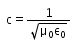Courses

# Test: Characteristics Of EM Waves

## 10 Questions MCQ Test Physics For JEE | Test: Characteristics Of EM Waves

Description
This mock test of Test: Characteristics Of EM Waves for JEE helps you for every JEE entrance exam. This contains 10 Multiple Choice Questions for JEE Test: Characteristics Of EM Waves (mcq) to study with solutions a complete question bank. The solved questions answers in this Test: Characteristics Of EM Waves quiz give you a good mix of easy questions and tough questions. JEE students definitely take this Test: Characteristics Of EM Waves exercise for a better result in the exam. You can find other Test: Characteristics Of EM Waves extra questions, long questions & short questions for JEE on EduRev as well by searching above.
QUESTION: 1

### The speed of electromagnetic waves in free space is given by. Here μ0 is ___________and ε0 is ________________ of free space.

Solution:

μ0 is called the permeability of free space and similarly, ε0 is called the permittivity of free space.

QUESTION: 2

### Which region in the electromagnetic spectrum will have the highest speed?

Solution:

All regions have the same speed.
The speed of a wave is not dependent upon its frequency and wavelength but rather upon the properties of the medium through which it travels.

QUESTION: 3

### Which region in the electromagnetic spectrum has the highest frequency?

Solution:

A gamma ray or gamma radiation is a penetrating electromagnetic radiation arising from the radioactive decay of atomic nuclei. It consists of the shortest wavelength electromagnetic waves and so imparts the highest photon energy

QUESTION: 4

Electromagnetic waves constitute of

Solution:

Maxwell predicted that an accelerated charge produces a sinusoidal time varying magnetic field, which in turn produces a sinusoidal time varying electric field. These two fields are mutually perpendicular to each other and sources of each other. They (fields) form an electromagnetic wave i.e. the wave that is propagated by simultaneous periodic variations of electric field and magnetic field intensity.

QUESTION: 5

In electromagnetic waves the ratio of amplitudes of electric field and magnetic field is equal to​

Solution:

In electromagnetic waves the ratio of amplitudes of electric field and magnetic field is equal to the velocity of the electromagnetic waves in free space.

(E/ B0) = c

QUESTION: 6

Which statement is true about electromagnetic waves?

Solution:

Properties:• Electromagnetic waves are propagated by oscillating electric and magnetic fields oscillating at right angles to each other. Hence (a) is incorrect.
• Electromagnetic waves travel with a constant velocity of  3 x 108 ms-1 in vacuum. Hence (c) is incorrect.
• Electromagnetic waves need no medium of propagation. The energy from the sun is received by the earth through electromagnetic waves. Hence, correct answer is (b).

QUESTION: 7

Which of the following is not a use of electromagnetic wave?

Solution:

Electromagnetic waves are not used to determine global warming.

QUESTION: 8

Which colour of the visible spectrum has the greatest wavelength?

Solution:

Red light has the lowest energy and is least scattered and has thus least frequency, thus we get that it has the largest wavelength. Similarly violet has maximum frequency.

QUESTION: 9

The electric field for an em wave is: E = E0sin[(12 x 1015 t – 4 x 107 x)]. What is the speed of the em waves?​

Solution:

V(velocity/speed)= ω/wave number (K)
V= 12×1015/4×107
V=3×108 m/s

QUESTION: 10

The wavelength of X-rays is of the order of:

Solution:

X-ray wavelength is in range of 10−11m to 10−8m

So option C is correct.